### 工程施工中，成都工程楼梯模板如何计算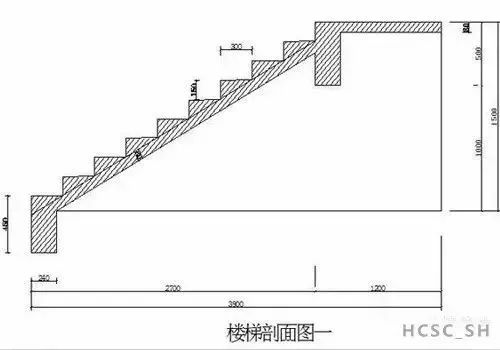（一）基本公式

（1）踏步高=150mm 踏步宽=300mm

（2）由勾股定理可得出踏步斜边长为：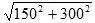=335.41mm

（3）坡度比例=短边/长边=150/300=0.5

（4）坡度系数=斜边/长边=335/300=1.118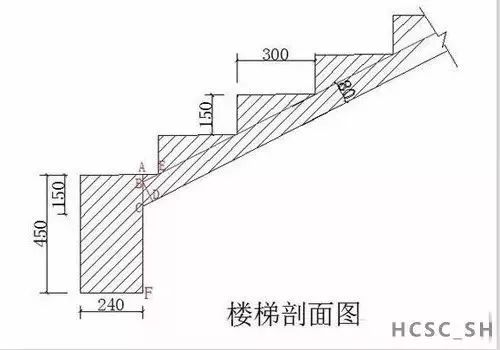（二）楼梯基础梁里侧模板的计算

AC=AB+BC

AE=300-240=60mm

AB=60×0.5=30mm

BC=80×1.118=90mm

AC=30+90=120mm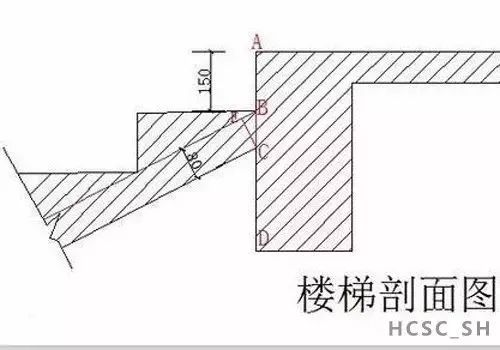(三)基础梁与平台梁..跑里侧模板的计算（楼梯剖面图三）

AC=AB+BC

AB=150mm

BC=80×1.118=90mm

CD=500-150-90=260mm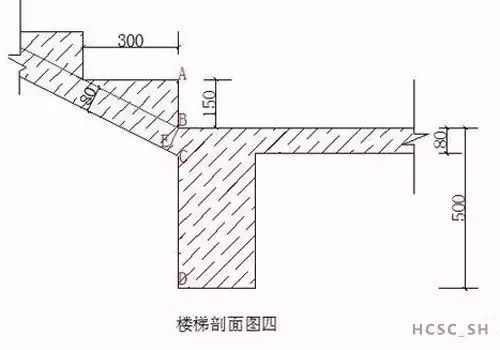（四）平台梁..跑与第二跑里侧模板的计算（楼梯剖面图四）

CD=BD-BC

BD=500mm

BC=80×1.118=90mm

CD=500-90=410mm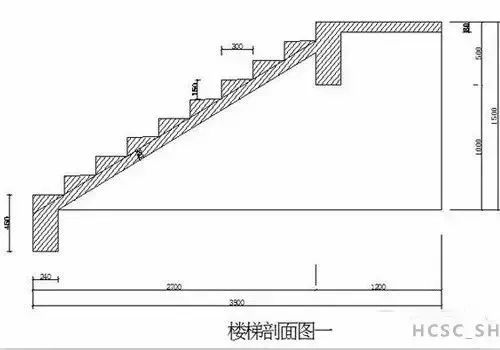（五）楼梯基础梁和..个平台梁连接底模板长度计算（可参照楼梯剖面图一）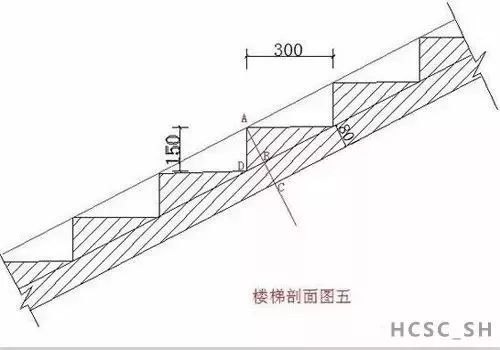（六）楼梯侧模（俗称楼梯帮高）计算（楼梯剖面图五）

AC=AB+BC

BC=80mm

AB=150÷1.118=134mm

AC=134+80=214mm

（七）附加先容楼梯支模合用工具之：三角板制作

【返回列表】

### 【推荐阅读】↓

Copyright   ©   2019 成都显高金属制品有限公司　版权所有 备案号：蜀ICP备19003361号-1   网站地图  RSS   XML    技术支持：